Courses

# Revisal Problems (Past 13 Year) JEE Advanced (D Block Elements)

## 30 Questions MCQ Test Chemistry for JEE Advanced | Revisal Problems (Past 13 Year) JEE Advanced (D Block Elements)

Description
This mock test of Revisal Problems (Past 13 Year) JEE Advanced (D Block Elements) for JEE helps you for every JEE entrance exam. This contains 30 Multiple Choice Questions for JEE Revisal Problems (Past 13 Year) JEE Advanced (D Block Elements) (mcq) to study with solutions a complete question bank. The solved questions answers in this Revisal Problems (Past 13 Year) JEE Advanced (D Block Elements) quiz give you a good mix of easy questions and tough questions. JEE students definitely take this Revisal Problems (Past 13 Year) JEE Advanced (D Block Elements) exercise for a better result in the exam. You can find other Revisal Problems (Past 13 Year) JEE Advanced (D Block Elements) extra questions, long questions & short questions for JEE on EduRev as well by searching above.
QUESTION: 1

### Only One Option Correct Type Direction (Q. Nos. 1-10) This section contains 10 multiple choice questions. Each question has four W o rk in g Space choices (a), (b), (c) and (d), out of which ONLY ONE is correct. Q.  Consider the four sulphates FeSO4, MnSO4, CoSO4 and ZnSO4, Four separate capsules of these materials have been made containing 0.1 mole of the substance and are suspended by a thread. Then a strong magnet is brought close to each capsule. Order of sulphates according to the strength of the response that the magnet will produce on these capsules is

Solution:

The substance which have unpaired electron shows paramagnetic behaviour. The number of unpaired electrons present in these substances as follows :
(d5)Mn2+ = 5 unpairede-
(d6)Fe2+ = 4 unpairede-
(d7)Co2+ = 3 unpairede-
(d10) Zn = no unpaired

QUESTION: 2

### A non-stoichiometric oxide of silver has composition Ag1.8O. What percentage of Ag is present in the atom of Ag2+?

Solution:

Consider one mole of the oxide, moles of M = 1.8 and moles of O2- = 1
Let moles of M2+ = x and moles of M+ = 1.8 - x
During charge balance
(1.8 - x) x 1 + 2x - 2 = 0
On solving, we get
x = 0.
Hence, percentage of M2+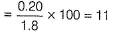QUESTION: 3

### Which of the following will not act as an oxidising agent?

Solution:

Stability of higher oxidation states increases down the group. WO3 is more stable in + 6. Hence, it does not act as an oxidising agent.

QUESTION: 4

Which of the following is the correct order of magnetic moment (in BM) for Mn2+, Cr2+ and V2+?

Solution:

The num ber of unpaired electrons in Mn2+, Cr2+ and V2+ are 5, 4 and 3, respectively.
The magnetic moment (BM) can be calculated by the spin-only formula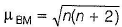where, n is the number of unpaired electrons.
Hence, the magnetic moment of Mn2+ ,Cr2+ and V2+ are 5.91,4.89 and 3.87 respectively.

QUESTION: 5

For a given sample of Fe2+ solution, if 30 mL of 0.1 volume of 0.1 M permanganate required is

Solution: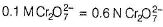and similarly, 0.1 M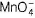= 0.5 NFe2+ =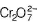== 30ML of 0.1M= V ML of 0.1 Mor 30 mL of 0.6 N= V mL of 0.5 MHence, volume of4 solution required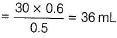QUESTION: 6

Which one of the following elements shows maximum number of different oxidation numbers in its compounds?

Solution:

Americium have +3, +4, +5 and +6 oxidation states.

QUESTION: 7

Oxidation state of mercury in amalgam, calomel and corrosive sublimate is

Solution:

Amalgam shows zero oxidation state, calomel (Hg2CI2)shows +1 oxidation state while corrosive sublimate (HgCI2)shows + 2 oxidation state.

QUESTION: 8

Which of the following electrode reactions has more negative SRP value?

Solution:

The SRP value is as follows :

Mn3+ / Mn2+ = + 1.57
Fe3+ / Fe2+ = + 0.77
Cr3+ / Cr2+ = - 0.41
V3+ / V2+ = -0.26

QUESTION: 9

Acidified K2Cr2O7 is treated with hydrogen sulphide. In this reaction, the oxidation state of chromium

Solution:

K2Cr2O7 + 4H2SO4 + 3H2S → K2SO+ Cr2(SO4)+ 7H2O + 3S

QUESTION: 10

Among the following strongest oxidising agent is:

Solution:

Among the given compound, [CrO4]2- show+6oxidation state which only reduces therefore, it is strongest reducing agent.

*Multiple options can be correct
QUESTION: 11

One or More than One Options Correct Type

Direction (Q. Nos. 11-20) This section contains 10 multiple choice questions. Each question has four choices (a), (b), (c) and (d), out of which ONE or MORE THAN ONE are correct.

Q.

Which of the following are soluble in water?

Solution:

AgBr is insoluble in water while AgF , AgNO3 and AgCIO4 are soluble in water.

*Multiple options can be correct
QUESTION: 12

Which are correct statements?

Solution:

The element which gives the greatest number of oxidation states occur in or near the middle of the series.

*Multiple options can be correct
QUESTION: 13

Which is correct regarding structure of dichromate ion?

Solution: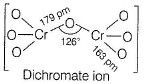*Multiple options can be correct
QUESTION: 14

Which are correctly matched ?

Solution:

Nessler’s reagent is a mixture of 2K2Hgl4 + 3KOH. It is used to test NH3(g) and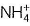.

*Multiple options can be correct
QUESTION: 15

Among the following metals, which have relatively higher second ionisation potential?

Solution:

Because second electron will remove from half-filled d-orbital in case of chromium and full-filled d-orbital in case of copper. Both these orbitals are more stable relative to other orbitals.

*Multiple options can be correct
QUESTION: 16

Find the correct statements.

Solution:

Metallic radii of zinc is greater than copper.
Zn (137 pm) > Cu (128 pm)

*Multiple options can be correct
QUESTION: 17

Which are correctly matched?

Solution:

MnO2 used in dry battery cells; TiO2 is used in pigm ent industry; Zeigler-Natta catalyst is AI(C2H5)+ TiCI4 and AgBr is used in photographic plates.

*Multiple options can be correct
QUESTION: 18

KMnO4 in an acidic medium oxidises.

Solution:

KMnO4 oxidises H2O2,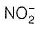and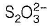in acidic medium.

*Multiple options can be correct
QUESTION: 19

Find incorrect statements.

Solution:

Cr shows highest oxidation state + 6. Mercury exists asHg+ and Hg2+ions.

*Multiple options can be correct
QUESTION: 20

The species having same number of unpaired electrons

Solution:

Eu2+, Gd3+ and Tb4+ have 7 unpaired electrons. Lu3+ have no unpaired electron.

QUESTION: 21

Comprehension Type

Direction (Q. Nos' 21 and 22) This section contains a paragraph, each describing theory, experiments, data, etc. Two questions related to the paragraph have been given. Each question has only one correct answer among the four given options (a), (b), (c) and (d).

Passage

A violet compound of manganese A decomposes on heating to give compounds 6 and C along with the liberation of a gaseous substance D. Compound C reacts with KOH in the presence of KNO3 to give compound B. On heating C with conc . H2SO4 and NaCI, greenish yellow gas E is liberated and a compound  of manganese F is formed along with other products.

Q.

The greenish yellow gas liberated in the above reactions is

Solution: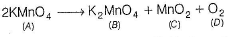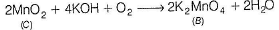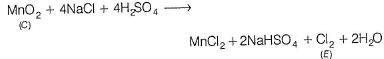QUESTION: 22

A violet compound of manganese A decomposes on heating to give compounds 6 and C along with the liberation of a gaseous substance D. Compound C reacts with KOH in the presence of KNO3 to give compound B. On heating C with conc . H2SO4 and NaCI, greenish yellow gas E is liberated and a compound  of manganese F is formed along with other products.

Q.

The compounds A and C in the above reactions are

Solution: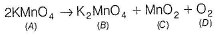Hence, A = KMnO4 and C = MnO2

QUESTION: 23

Matching List Type

Direction (Q. Nos.23 and 24) Choices for the correct combination of elements from Column I and Column II are given as options (a), (b), (c) and (d), out of which one is correct.

Q.

Match the following reagents given in Column I with the Column II. Mark the correct option from the codes given below.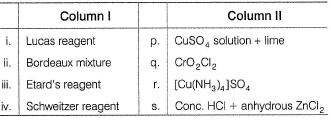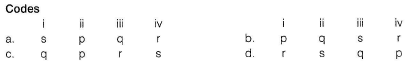Solution:

(i) → (s),(ii) → (p).(iii) → (q) ,(iv) → (r)

QUESTION: 24

Match the following characteristics given in Column I with the corresponding elements given in Column II. Mark the correct option from the codes given below.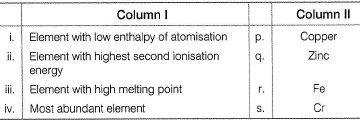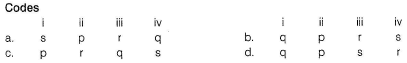Solution:

(i) → (q), (ii) (p), (iii) → (s), (iv) → (r)

*Answer can only contain numeric values
QUESTION: 25

One Integer Value Correct Type

Direction (Q. Nos. 25-30) This section contains 6 questions. When worked out will result in an integer from 0 to 9 (both inclusive).

Q.

What is van't Hoff factor if Hg2(NO3)2 is 100% ionised in aqueous solution?

Solution: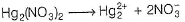*Answer can only contain numeric values
QUESTION: 26

The number of electrons in 4f subshell of +4 oxidation state of terbium are

Solution:

Tb = [Xe] 4795cf°6s2 andTb4+ = [Xe] 4775c7°6s°

*Answer can only contain numeric values
QUESTION: 27

The calculated magnetic moment of Fen+ ion is 4.90 BM. The sum of the number of electrons presemn 3d subshell of Fen+ ion and the value of n is

Solution:

Magnetic moment 4.90 BM indicates that Fe has 4 unpaired electrons. Then iron is Fe2+.

*Answer can only contain numeric values
QUESTION: 28

In solid CuSO4.5H2O copper is coordinated to ......... water molecules.

Solution:

In solid CuSO4-5H2O, copper is coordinated to 4 water molecules.

*Answer can only contain numeric values
QUESTION: 29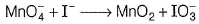when this equation is balanced in basic medium, the sum of the coefficients in the balanced equation is

Solution: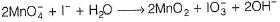*Answer can only contain numeric values
QUESTION: 30

Among the following ion, the number of ions which are colourless

La3+, Pr3+,Lu3+,Ce3+,Tm3+,Yb3+,Er3+

Solution:

La3+ - colourless, Pr3+- yellow green Lu3+ - colourless, Ce3+ - colourless

Tm3+ - green, Yb3+ - colourless, Er3+ - green